# Recreating a Geometric CDF plot from Casella and Berger

For reasons I no longer remember I decided a while back to reproduce Figure 1.5.2 from Casella and Berger (page 32):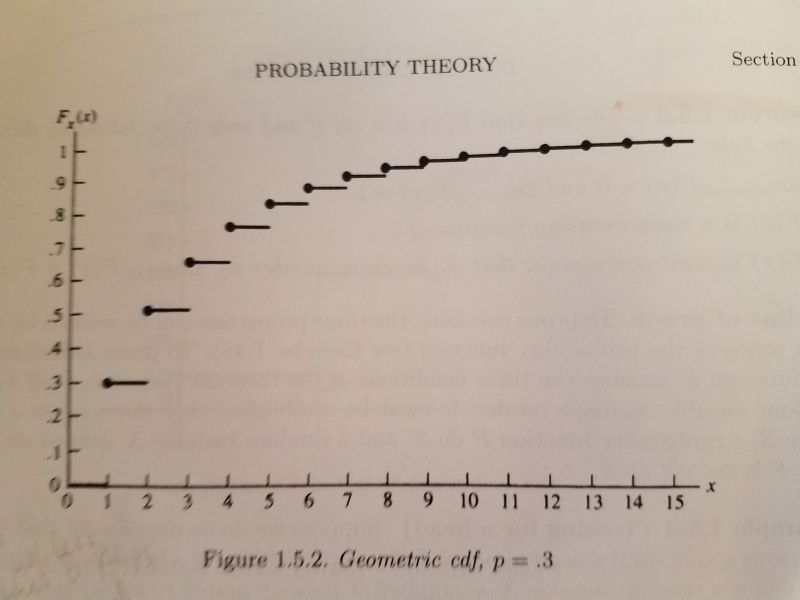It's a plot of the cumulative distribution function of a geometric distribution with p = 0.3. The geometric distribution is best explained with coin-flipping. Let's say we keep flipping a coin until we observe a head. Now let X be a random variable that equals the number of tosses required to see a head. So if we get a head on the first flip of the coin, that means we needed one toss. If it takes two tosses, we got a tail on the first toss and then a head on the second toss. The graph above visualizes the possibilities for 15 flips with probability of getting heads set to 0.3. We see that the probability of taking only one toss is 0.3, which makes sense since the probability of getting heads is 0.3. The probability of requiring two tosses is about 0.5. And so on. The straight lines indicate the probabilities only change at the whole numbers. There is no such thing as, say, 2.23 flips. This is often referred to as a step function. We see that there's a high probability of getting a head by the 5th or 6th flip. This is a simple waiting-time distribution that can be used to model the number of successes before a failure and vice-versa.

To recreate this plot in R I used the pgeom function, which returns the cumulative probability of the geometric distribution for a given number of failures before a success occurs and a given probability of success. For example, the probability of 0 failures before observing a success with p = 0.3:

pgeom(0, prob = 0.3)

##  0.3


The probability of 1 failure before observing a success with p = 0.3:

pgeom(1, prob = 0.3)

##  0.51


To generate all the probabilities in the plot, we can do the following:

y <- pgeom(q = 0:14, prob = 0.3)


Notice that instead of using 1:15 (number of total flips for success), we use 0:14. That's because pgeom works with the number of failures before success. Now that we have our y coordinates, we can create our first version of the plot as follows:

plot(x = 1:15, y, pch = 19)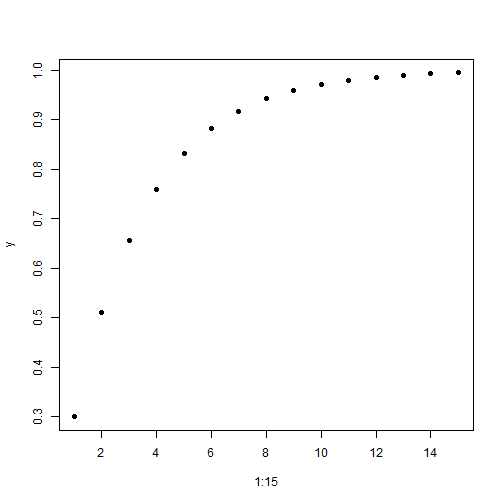Now let's add the horizontal lines using the segments function:

plot(x = 1:15, y, pch = 19)
segments(x0 = 0:15, y0 = c(0, y),
x1 = 1:16, y1 = c(0, y), lwd = 2)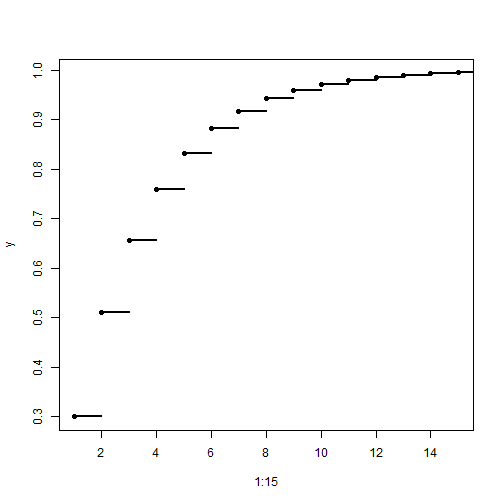The x0 and y0 coordinates are where the line starts. The x1 and y1 coordinates are where the line ends. Since the lines are horizontal, the y coordinates are the same for the start and end postions. The y coordinates include 0, so we add that value to y with the c function. The lwd = 2 argument makes the line a little thicker and darker.

Our plot is basically done, but just for fun I wanted to see how close I could make it look like the version in the book. That means relabeling the axes, moving the axis labels to the ends, and removing the lines at the top and right side of the plot. It also means moving the axis tick marks inside the plotting area. After some trial and error and deep dives into R documentation, here's what I was able to come up with:

plot(x = 1:15, y, pch = 19,
yaxt = "n", xaxt = "n", ylim = c(0,1.05), xlim = c(0,15.5),
bty="l", xaxs="i", yaxs = "i", xlab = "", ylab = "")
segments(x0 = 0:15, y0 = c(0, y),
x1 = 1:16, y1 = c(0, y), lwd = 2)
axis(side = 1, at = 0:15,
labels = 0:15, tcl = 0.5, family = "serif")
axis(side = 2, at = seq(0,1,0.1),
labels = c(0,paste(".",1:9),1), las=1, tcl = 0.5, family = "serif")
mtext(text = expression(italic(x)), side = 4,
at = 0, las = 1, line = 0.5, family = "serif")
mtext(text = expression(italic(F[x](x))), side = 3,
at = 0, line = 0.5, family = "serif")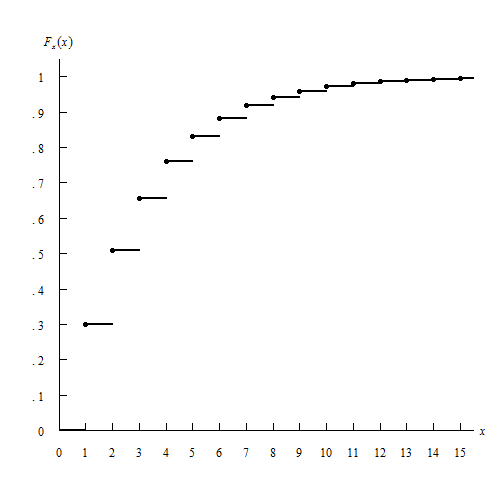In the plot function:

• the yaxt = "n" and xaxt = "n" arguments say “don't label the axes”. I instead use the axis function to create the axes.
• the ylim = c(0,1.05) and xlim = c(0,15.5) arguments tell the axes to end at 1.05 and 15.5, respectively. I wanted them to extend beyond the last value just as they do in the book.
• the bty="l" argument says “draw a box around the plot like a capital letter L”
• the xaxs="i" and yaxs = "i" arguments ensures the axes fit within the original range of the data. The default is to extend the range by 4 percent at each end. Again, I was trying to recreate the graph in the book. Notice how the origin has the x-axis and y-axis 0 values right next to one another.
• The xlab = "" and ylab = "" set blank axis labels. I instead use the mtext function to add axis labels.

The segments function remained unchanged.

The axis function allows us to explicitly define how the axis is created.

• The side argument specifies which side of the plot we're placing the axis. 1 is the bottom, 2 is the left.
• at is where we draw the tick marks.
• labels are how we label the tick marks.
• The tcl argument specifies how long to make the tick marks and in what direction. A positive value extends the tick marks into the plotting region.
• The las argument in the second axis function makes the labels on the y-axis horizontal.

Finally I used the mtext function to create the axis labels. mtext writes text into the margins of a plot and can take some trial and error to get the placement of text just so.

• The text argument is what text we want to place in the graph. In this case I make use of the expression function which allows us to create mathematical notation. For example, the syntax expression(italic(F[x](x))) returns $$F_x (x)$$
• The side argument again refers to where in the plot to place the text. 3 is top and 4 is right. This means the y-axis label is actually in the top of the plot and the x-axis label is on the right. A little bit of a hack.
• at says where to place the text along the axis parallel to the margin. In both cases we use 0. We want the y-axis label at the 0 point corresponding to the x-axis, and the x-axis label at the 0 point corresponding to the y-axis. A little confusing, I think.
• The las argument rotates the x label to be horizontal
• The line argument specifies on which margin line to place the text, starting at 0 counting outwards. This is one that takes some trial and error to get just right.
• The family argument specifies the type of font to use. “serif” is like Times New Roman.

Not perfect, but close enough. Of course I much prefer the R defaults when it comes to plotting layout. Even though R allows us to recreate this graph, I don't think it's necessarily a “good” graph.

I also decided to tackle this using ggplot. Here's how far I got.

library(ggplot2)
dat <- data.frame(x = 1:15, y = pgeom(q = 0:14, prob = 0.3))
dat2 <- data.frame(x = 0:15, y = c(0, dat$y[-16]), xend = 1:16, yend = c(0,dat$y[-16]))

ggplot(dat, aes(x = x, y = y)) + geom_point() +
geom_segment(aes(x = x, y = y, xend = xend, yend = yend), data = dat2) +
scale_x_continuous(breaks = 0:15, labels = 0:15) +
scale_y_continuous(breaks = seq(0,1,0.1), labels = c(0,paste(".",1:9),1)) +
labs(x = "x", y = expression(italic(F[x](x)))) +
theme(panel.background = element_blank(),
axis.line.x = element_line(color = "black"),
axis.line.y = element_line(color = "black"),
axis.title.x = element_text(face = "italic", hjust = 1),
axis.title.y = element_text(face = "italic", hjust = 1, angle = 0))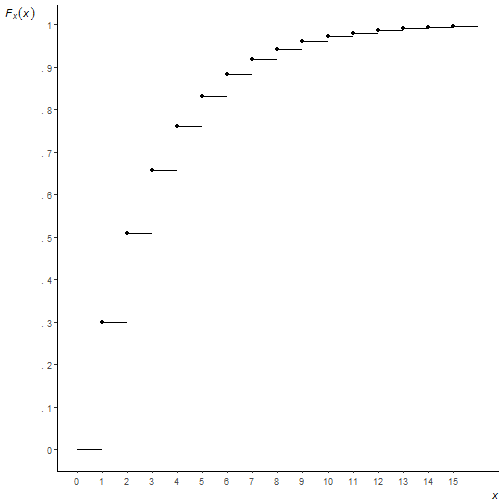You can see I couldn't figure out how to move the axis ticks into the plotting region, or how to place axis labels at the ends of the axis, or how to get the origin to start at precisely (0, 0). I'm not saying it can't be done, just that I lost interest in trying to go further. And a very strong argument can be made that these are things you shouldn't do anyway! But as I said at the outset, this was all for fun.

This site uses Akismet to reduce spam. Learn how your comment data is processed.